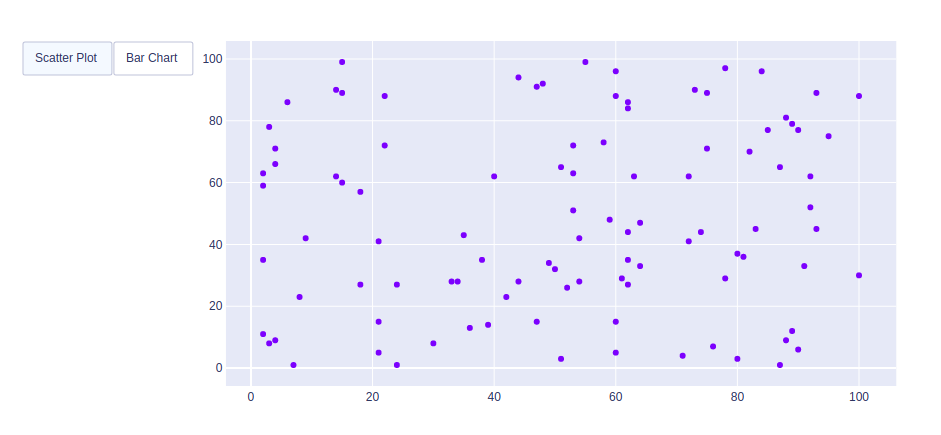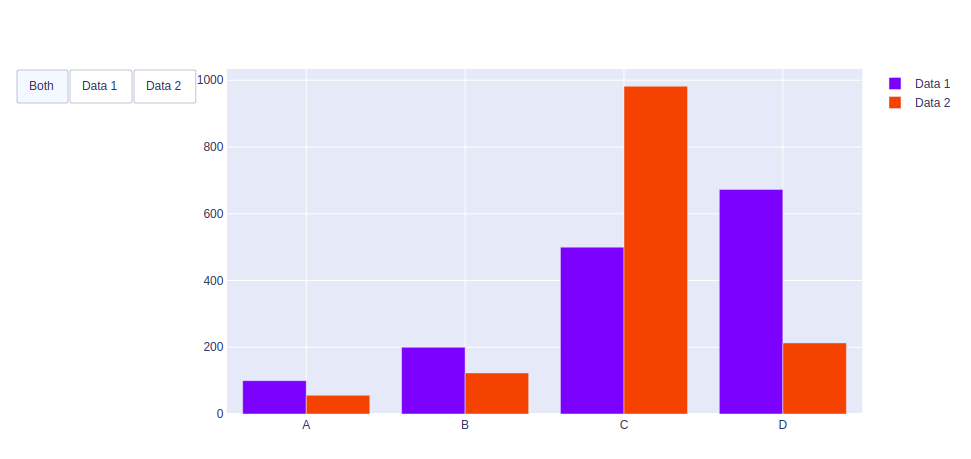# How to make Custom Buttons in Plotly?

A Plotly is a Python library that is used to design graphs, especially interactive graphs. It can plot various graphs and charts like histogram, barplot, boxplot, spreadplot, and many more. It is mainly used in data analysis as well as financial analysis. plotly is an interactive visualization library.

## Making Custom Buttons

In plotly, actions custom Buttons are used to quickly make actions directly from a record. Custom Buttons can be added to page layouts in CRM, Marketing, and Custom Apps. There are 4 possible methods that can be applied in custom buttons:

• restyle: modify data or data attributes
• relayout: modify layout attributes
• update: modify data and layout attributes
• animate: start or pause an animation

Example 1: Using Restyle Method

## Python3

 `import` `plotly.graph_objects as px ` `import` `numpy as np ` ` `  ` `  `# creating random data through randomint ` `# function of numpy.random ` `np.random.seed(``42``) ` ` `  `random_x ``=` `np.random.randint(``1``, ``101``, ``100``) ` `random_y ``=` `np.random.randint(``1``, ``101``, ``100``) ` ` `  `plot ``=` `px.Figure(data``=``[px.Scatter( ` `    ``x``=``random_x, ` `    ``y``=``random_y, ` `    ``mode``=``'markers'``,) ` `]) ` ` `  `# Add dropdown ` `plot.update_layout( ` `    ``updatemenus``=``[ ` `        ``dict``( ` `            ``type``=``"buttons"``, ` `            ``direction``=``"left"``, ` `            ``buttons``=``list``([ ` `                ``dict``( ` `                    ``args``=``[``"type"``, ``"scatter"``], ` `                    ``label``=``"Scatter Plot"``, ` `                    ``method``=``"restyle"` `                ``), ` `                ``dict``( ` `                    ``args``=``[``"type"``, ``"bar"``], ` `                    ``label``=``"Bar Chart"``, ` `                    ``method``=``"restyle"` `                ``) ` `            ``]), ` `        ``), ` `    ``] ` `) ` ` `  `plot.show() `

Output:Example 2: Using Update method

## Python3

 `import` `plotly.graph_objects as px ` `import` `numpy ` ` `  ` `  `# creating random data through randomint ` `# function of numpy.random ` `np.random.seed(``42``) ` ` `  `random_x ``=` `np.random.randint(``1``, ``101``, ``100``) ` `random_y ``=` `np.random.randint(``1``, ``101``, ``100``) ` ` `  `x ``=` `[``'A'``, ``'B'``, ``'C'``, ``'D'``] ` ` `  `plot ``=` `px.Figure(data``=``[go.Bar( ` `    ``name``=``'Data 1'``, ` `    ``x``=``x, ` `    ``y``=``[``100``, ``200``, ``500``, ``673``] ` `), ` `    ``go.Bar( ` `    ``name``=``'Data 2'``, ` `    ``x``=``x, ` `    ``y``=``[``56``, ``123``, ``982``, ``213``] ` `) ` `]) ` ` `  ` `  `# Add dropdown ` `plot.update_layout( ` `    ``updatemenus``=``[ ` `        ``dict``( ` `            ``type``=``"buttons"``, ` `            ``direction``=``"left"``, ` `            ``buttons``=``list``([ ` `                ``dict``(label``=``"Both"``, ` `                     ``method``=``"update"``, ` `                     ``args``=``[{``"visible"``: [``True``, ``True``]}, ` `                           ``{``"title"``: ``"Both"``}]), ` `                ``dict``(label``=``"Data 1"``, ` `                     ``method``=``"update"``, ` `                     ``args``=``[{``"visible"``: [``True``, ``False``]}, ` `                           ``{``"title"``: ``"Data 1"``, ` `                            ``}]), ` `                ``dict``(label``=``"Data 2"``, ` `                     ``method``=``"update"``, ` `                     ``args``=``[{``"visible"``: [``False``, ``True``]}, ` `                           ``{``"title"``: ``"Data 2"``, ` `                            ``}]), ` `            ``]), ` `        ``) ` `    ``]) ` ` `  `plot.show() `

Output:Whether you're preparing for your first job interview or aiming to upskill in this ever-evolving tech landscape, GeeksforGeeks Courses are your key to success. We provide top-quality content at affordable prices, all geared towards accelerating your growth in a time-bound manner. Join the millions we've already empowered, and we're here to do the same for you. Don't miss out - check it out now!